# Cholesky factorization

Jump to: navigation, search

A symmetric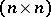matrix(cf. also Symmetric matrix) is positive definite if the quadratic formis positive for all non-zero vectorsor, equivalently, if all the eigenvalues ofare positive. Positive-definite matrices have many important properties, not least that they can be expressed in the form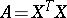for a non-singular matrix. The Cholesky factorization is a particular form of this factorization in whichis upper triangular with positive diagonal elements, and it is usually written as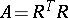. In the case of a scalar (), Cholesky factorization corresponds to the fact that a positive number has a positive square root. The factorization is named after A.-L. Cholesky, a French military officer involved in geodesy. It is closely connected with the solution of least-squares problems (cf. also Least squares, method of), since the normal equations that characterize the least-squares solution have a symmetric positive-definite coefficient matrix.

The Cholesky factorization can be computed by a form of Gaussian elimination that takes advantage of the symmetry and definiteness. Equatingelements in the equationgives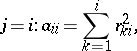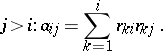These equations can be solved to yielda column at a time, according to the following algorithm:

<tbody> </tbody>
 FOR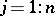FOR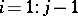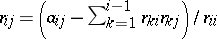ENDEND

It is the positive definiteness ofthat guarantees that the argument of the square root in this algorithm is always positive.

It can be shown [a1] that in floating-point arithmetic the computed solution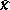satisfies, where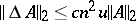, witha constant of orderandthe unit round-off (or machine precision). Here,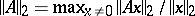and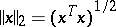. This excellent numerical stability is essentially due to the equality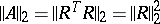, which guarantees thatis of bounded norm relative to.

A variant of Cholesky factorization is the factorization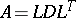whereis unit lower triangular andis diagonal. This factorization exists for definite matrices and some (but not all) indefinite ones. Whenis positive definite, the Cholesky factor is given by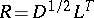.

A symmetric matrixis positive semi-definite if the quadratic formis non-negative for all; thus,may be singular (cf. also Matrix). For such matrices a Cholesky factorization exists, butmay not display the rank of. However, by introducing row and column permutations it is always possible to obtain a factorizationwhereis a permutation matrix,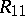isupper triangular with positive diagonal elements, and.

Cf. Matrix factorization for more details and references.